RS Aggarwal Class 7 Solutions Chapter 10 - Percentage

RS Aggarwal Class 7 Chapter 10 - Percentage Solutions Free PDF

A mathematical number or ratio, which is expressed as a fraction of 100 is called Percentage. It can be represented as ‘%’ and it is a dimensionless quantity. It is an important mathematical function, that can be used to finding a certain percentage from a sample. Some of the different percent changes are either a percentage increase or percentage decrease.

Some other different percentage concepts that are introduced in this chapter are:

1. Mass percent
2. Mole percent
3. Percentage point
4. Percentage difference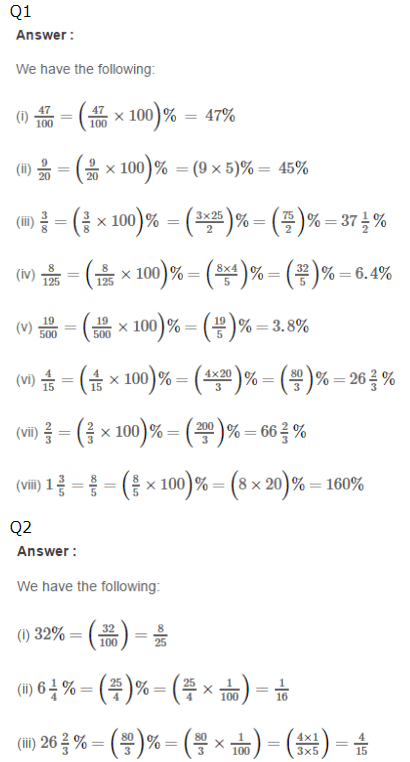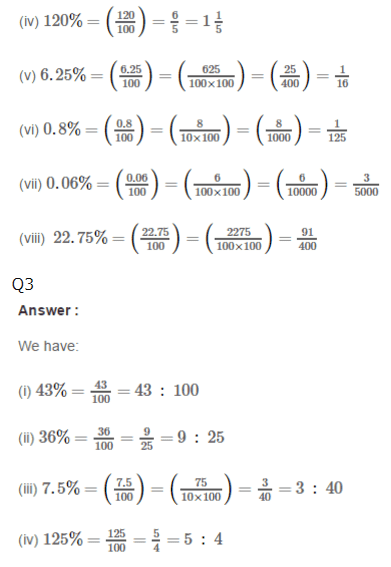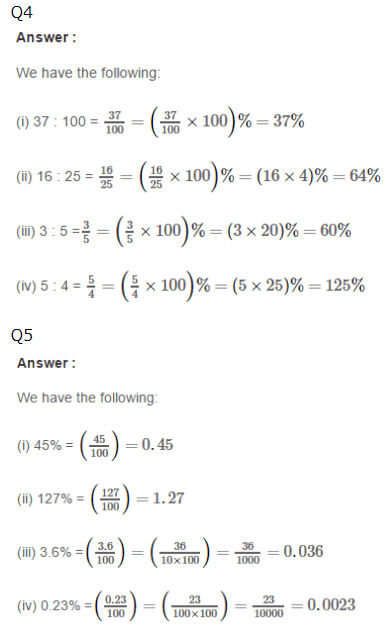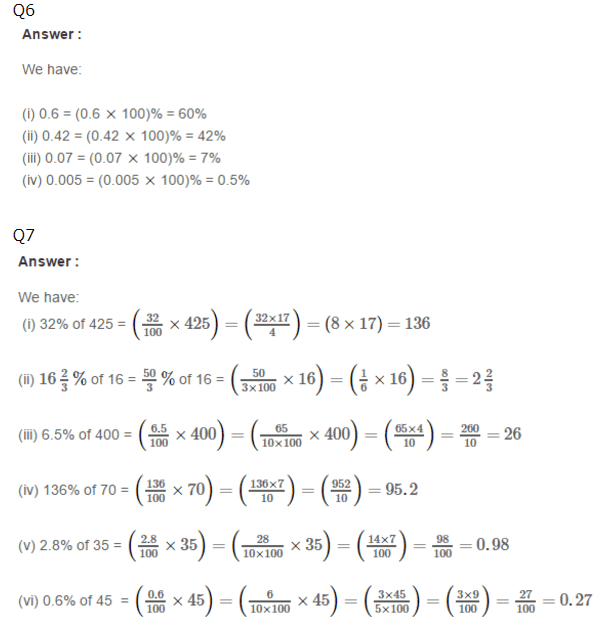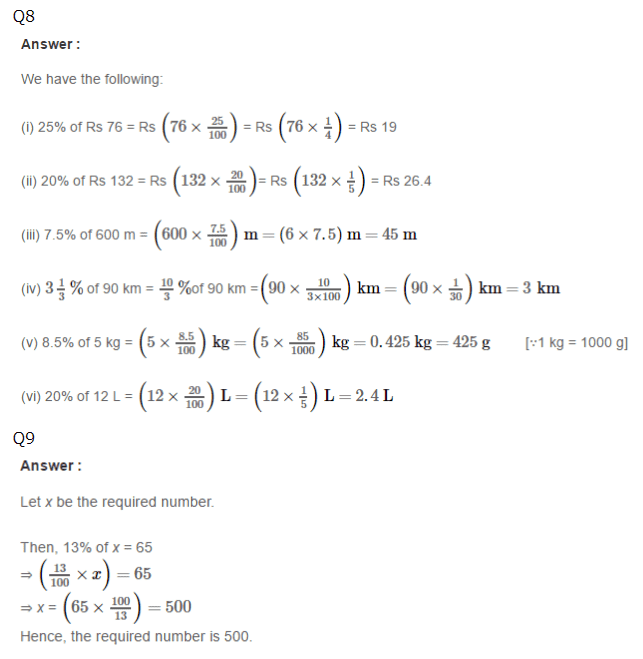Practise This Question

Electric bell works on which of the following effects of current?